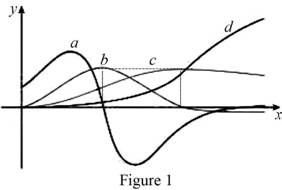# Each curves on the given graph and give proper explanation.### Single Variable Calculus: Concepts...

4th Edition
James Stewart
Publisher: Cengage Learning
ISBN: 9781337687805### Single Variable Calculus: Concepts...

4th Edition
James Stewart
Publisher: Cengage Learning
ISBN: 9781337687805

#### Solutions

Chapter 2.7, Problem 44E
To determine

## To identify: Each curves on the given graph and give proper explanation.

Expert Solution

In the given graph, d is position curve, c is velocity curve, b is acceleration curve and a is the jerk curve.

### Explanation of Solution

Note: Let f and f be the graph of a function and its derivative on the same screen. Then f(a)=0 where a is x-coordinate of the point where f has horizontal tangent.

Graph:

The given graph is shown as in Figure 1,Observation:

From Figure 1, the point where a(t)=0 is the same point where graph of b(t) has horizontal slope.

Recall that the derivative of a function is zero where the function has a horizontal tangent.

The curve a(t) is the derivative of the graph b(t).

Thus, b(t)=a(t). (1)

Observe the graph of b and c carefully.

The point where b(t)=0 is the same point where graph of c(t) has horizontal slope.

Recall that the derivative of a function is zero where the function has a horizontal tangent.

The curve b(t) is the derivative of the graph c(t).

Thus, c(t)=b(t). (2)

From (1) and (2), b(t)=a(t) and c(t)=b(t). This implies that, b(t)=c(t).

There are two possibilities: c(t)=d(t) or d(t)=a(t)

Observe the graph of d and a carefully.

The points where the graph of a has horizontal tangents, the functional value of d is not zero at that points.

This implies that a(t)d(t).

The only possibility is that a is the acceleration curve.

This implies c(t)=d(t).

Thus d is position curve, c is velocity curve, b is acceleration curve and a is the jerk curve.

### Have a homework question?

Subscribe to bartleby learn! Ask subject matter experts 30 homework questions each month. Plus, you’ll have access to millions of step-by-step textbook answers!